Scores are an Advanced Logic feature available on all Typeform plans. Scores are also available as a variable on all typeforms. This walkthrough goes over how you can use Logic to add scores to answers when creating quizzes, lead qualification forms, shopping baskets or order forms.

Using a Payment question? You may want to use the price variable instead of score - read this article to find out more.

1. Here is a Number question, asking how many of something someone wants.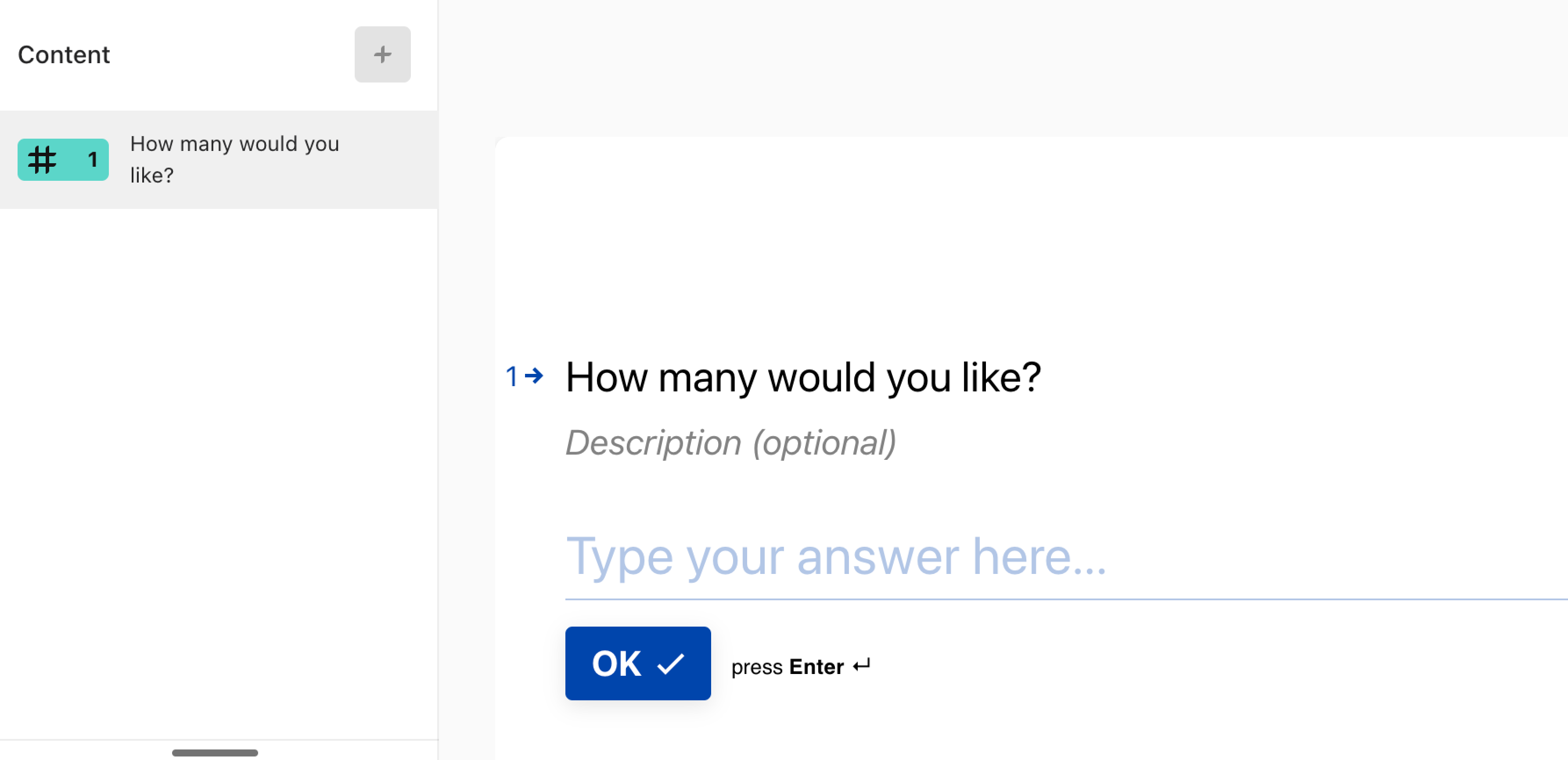Let's say each unit costs \$5, so I want the answer to this question to be multiplied by 5.

Click Logic in the right-hand panel, then Branching and calculations: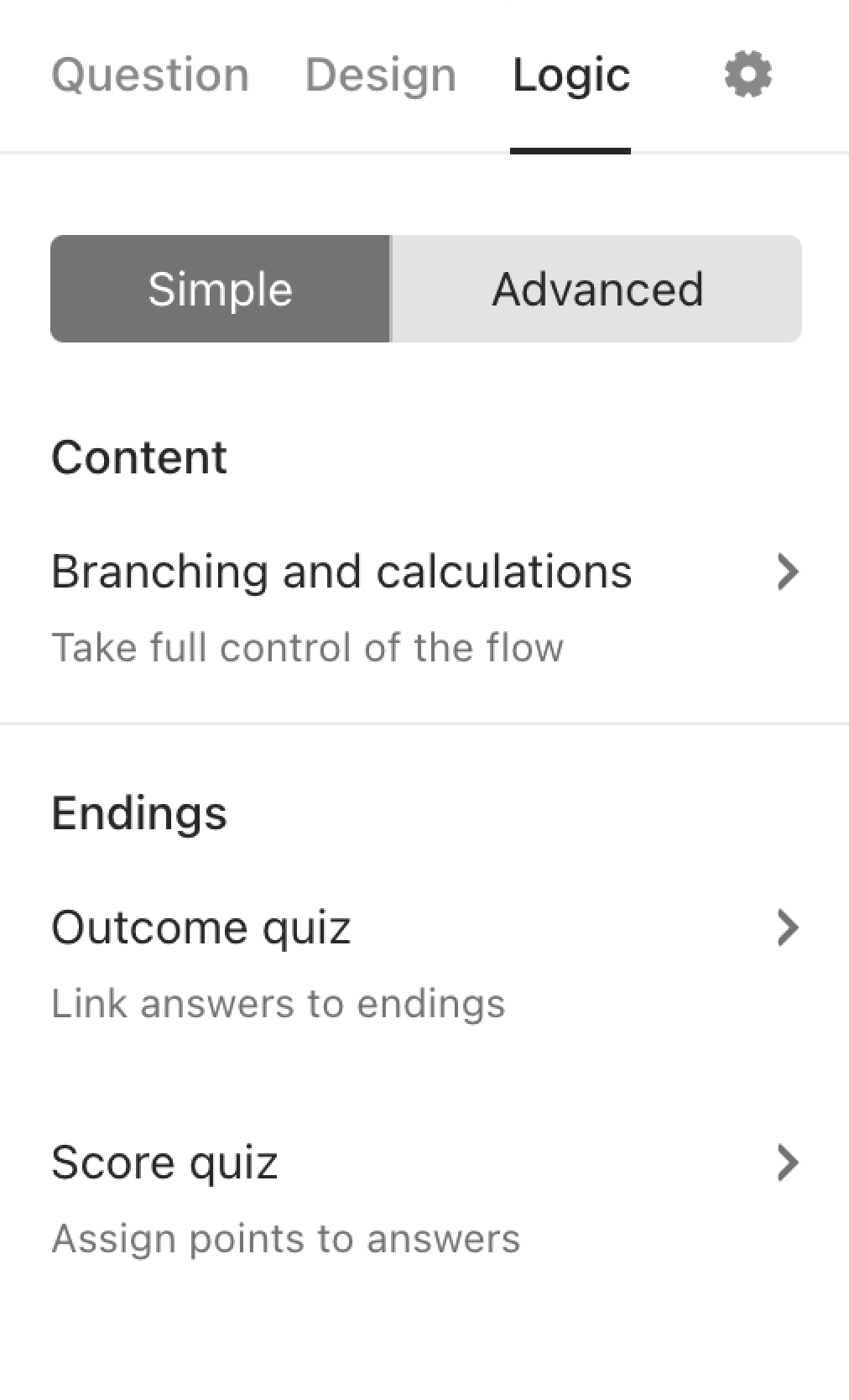2. A new window will open: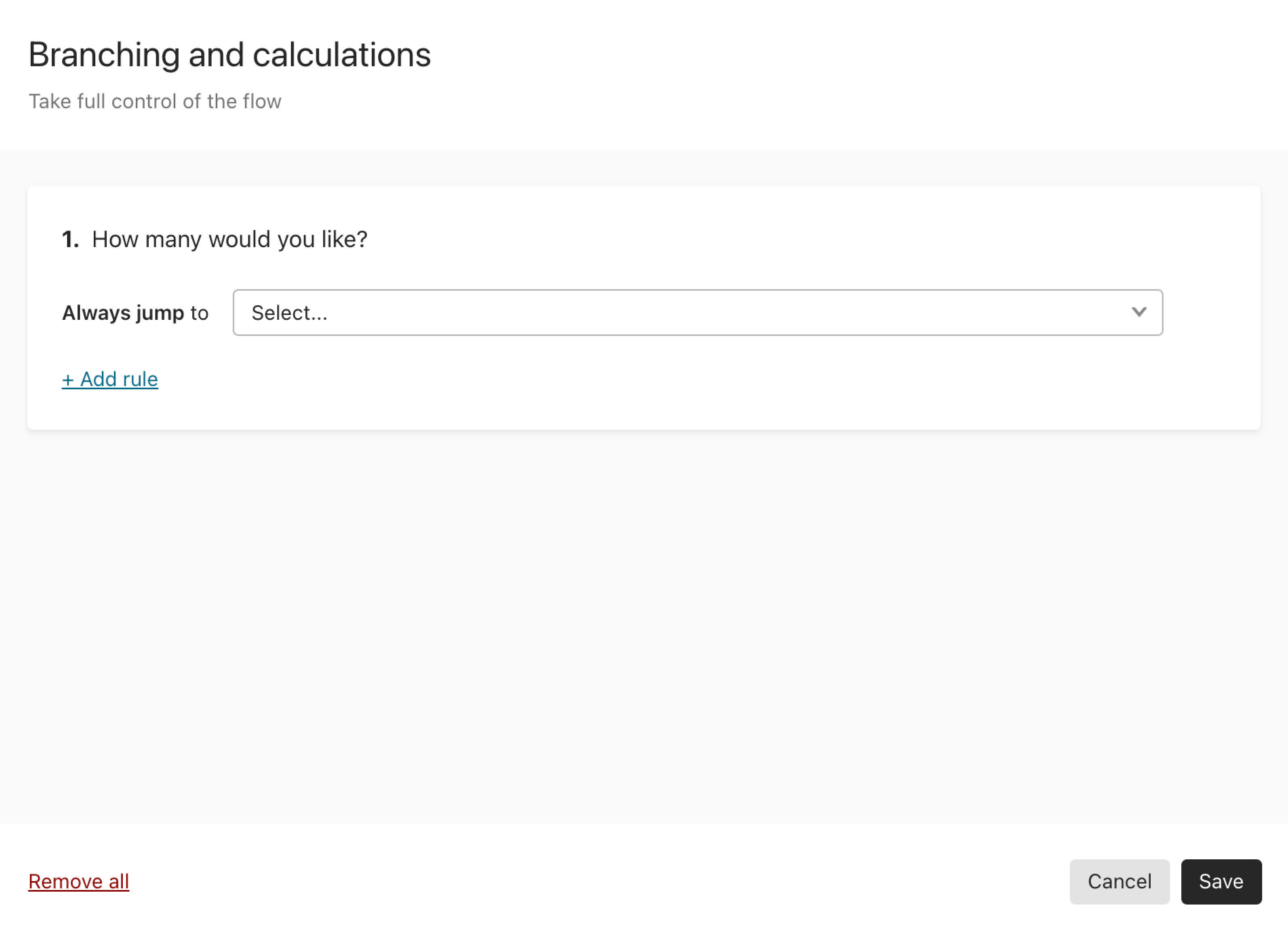3. Now click the If dropdown menu, and select Always.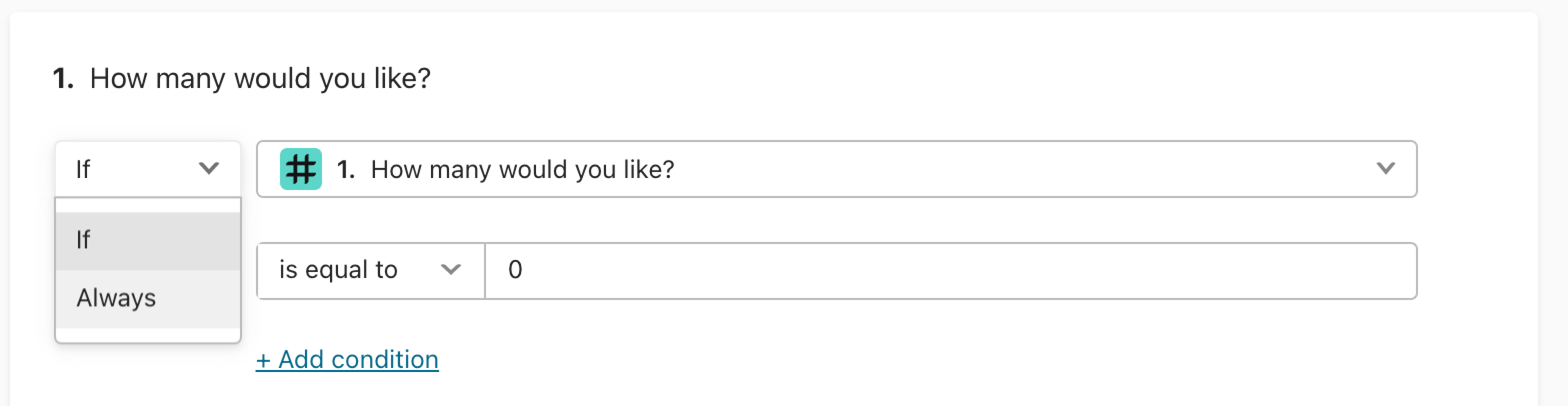4. Now click the Select... dropdown menu, which will show the calculation functions available: Add, Divide, Subtract or Multiply.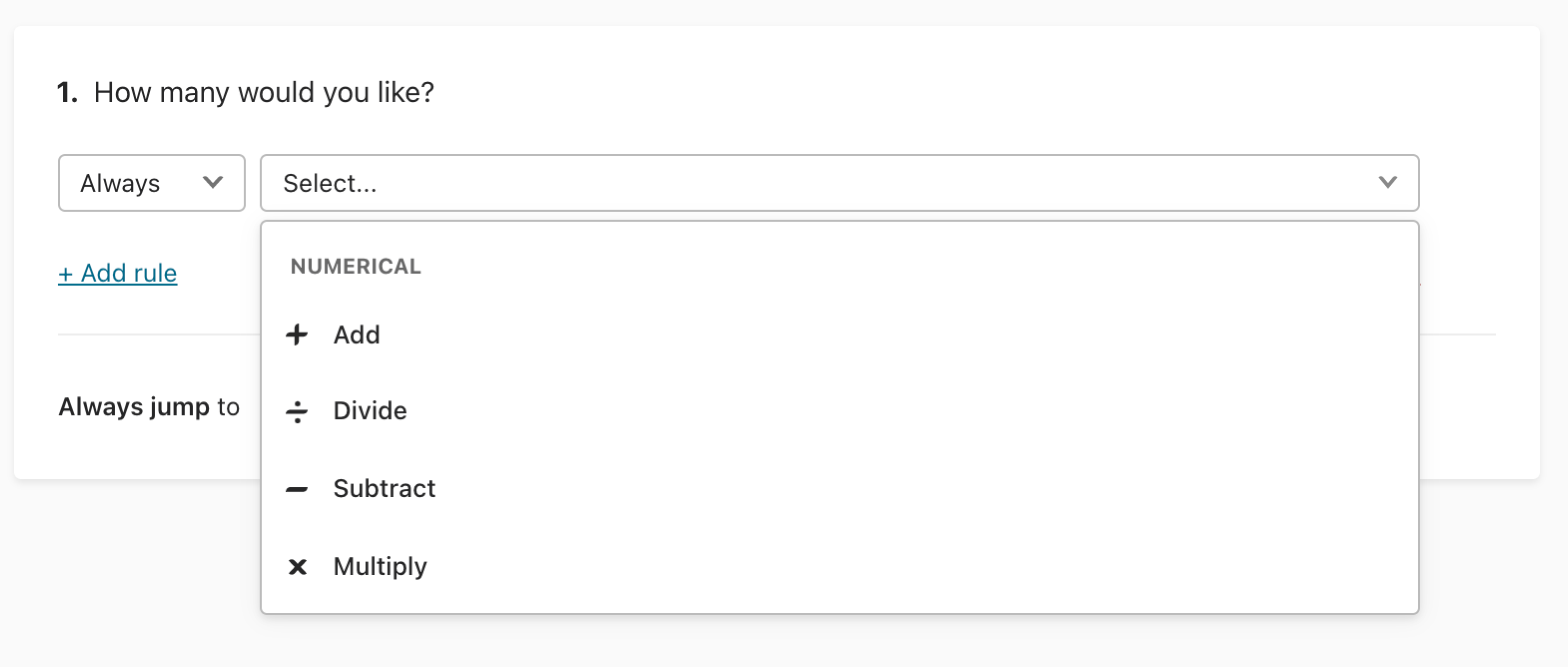Here we'll choose Multiply. Now we can choose our multiplication from the drop down menus. We will multiply the score variable by the answer to the question "How many would you like?".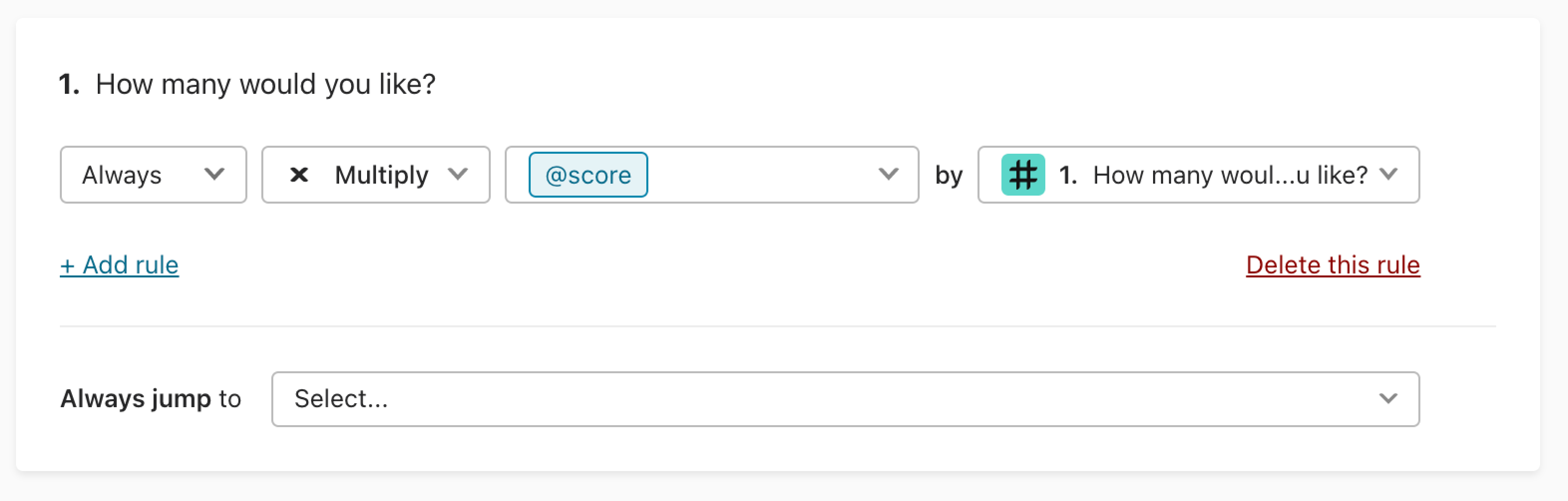Click Save to finish setting up your calculation.

5. You can also choose to Divide, which looks like this: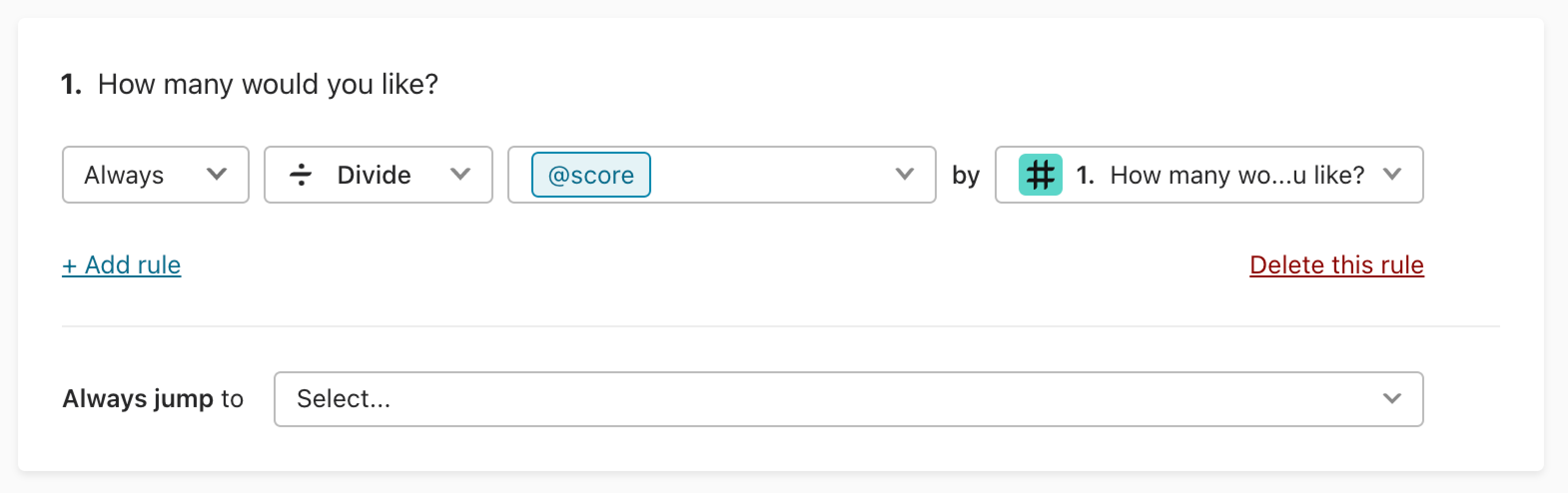6. When you choose Add or Subtract, the calculation changes as follows: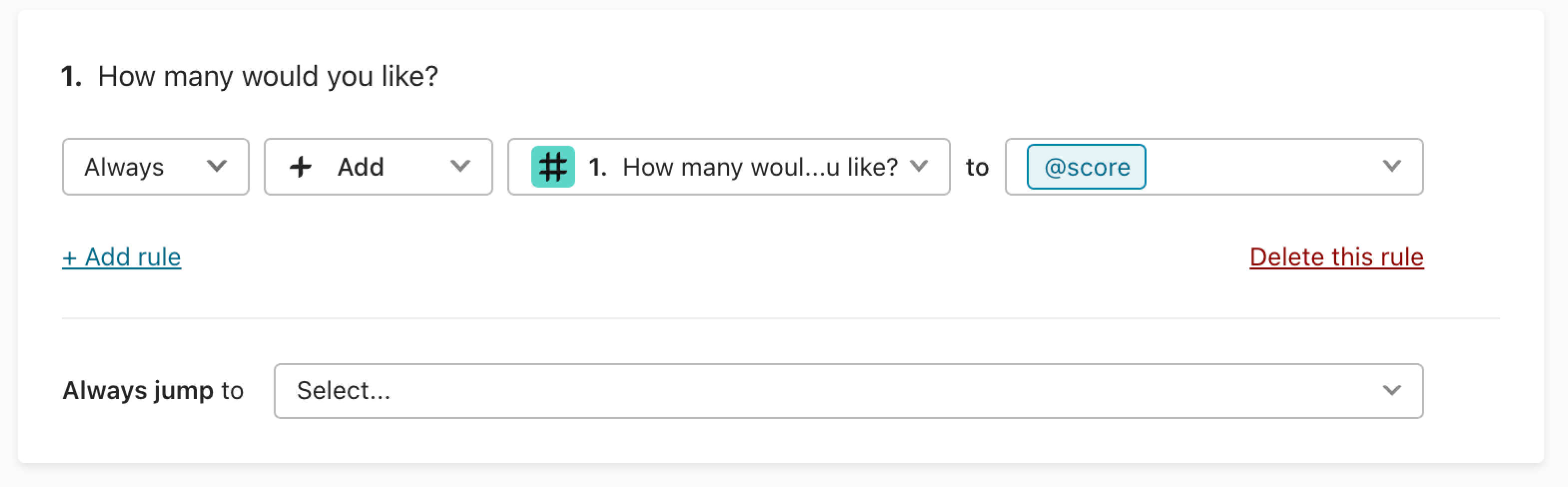######Note! Calculations with Always conditions are executed when the typeform loads, not when respondents reach a question with calculations. For example, if you choose to always add an amount to the price variable, the variable is populated before respondents answer the given question. To avoid this, we recommend adding If conditions to calculations. This way the calculations are only executed when respondents reaches the question.

7. Changing the Always dropdown to If lets you create calculations for specific answers.

For example, here, if someone answers 10, I want to add 7 to the score. I might do this if I want to give people a discount for buying larger amounts, for example.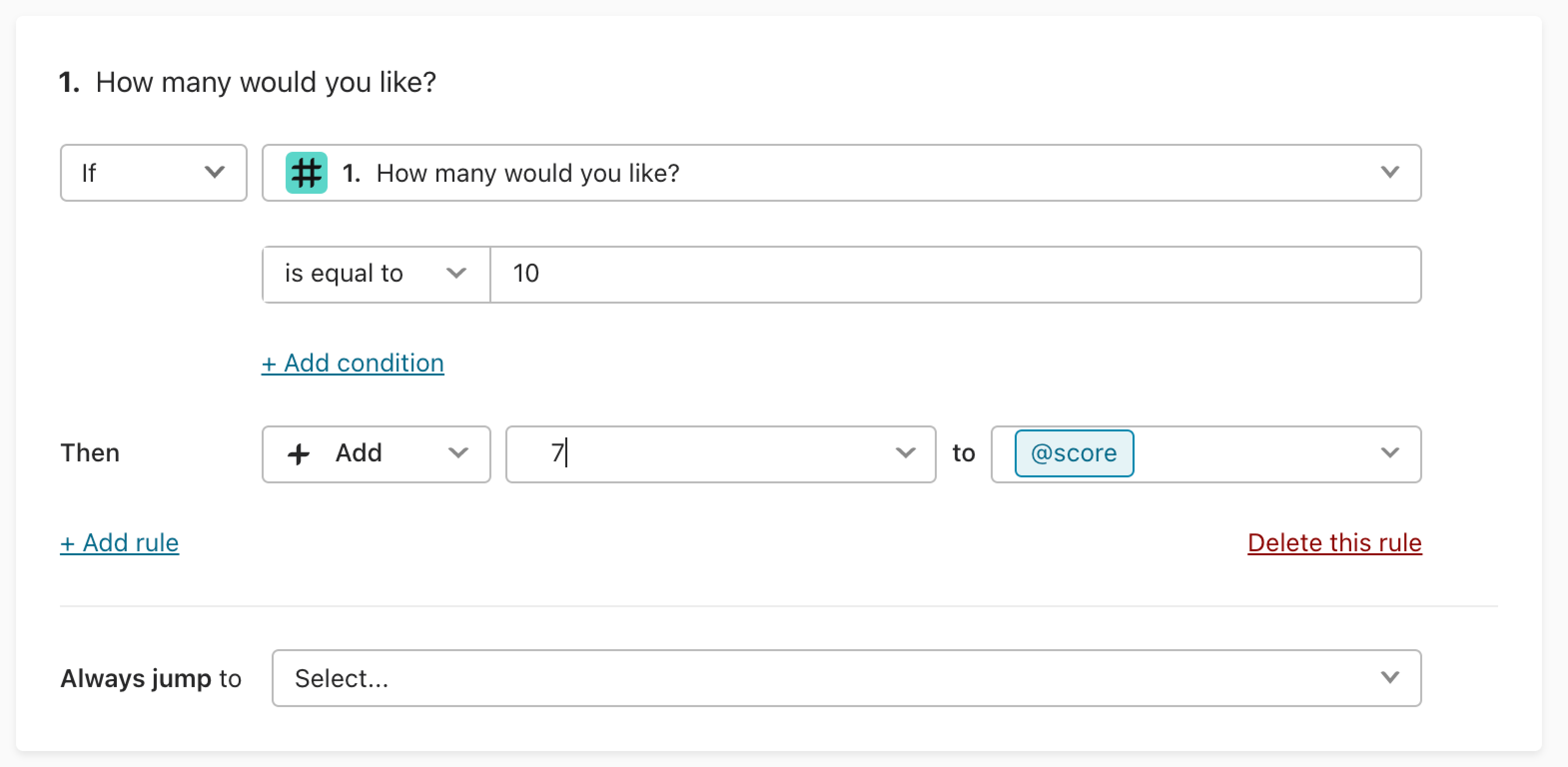You can also apply calculation-based rules to other question types. For example, here is a Multiple Choice question. If someone chooses Car, then I want to add 50,000 to score: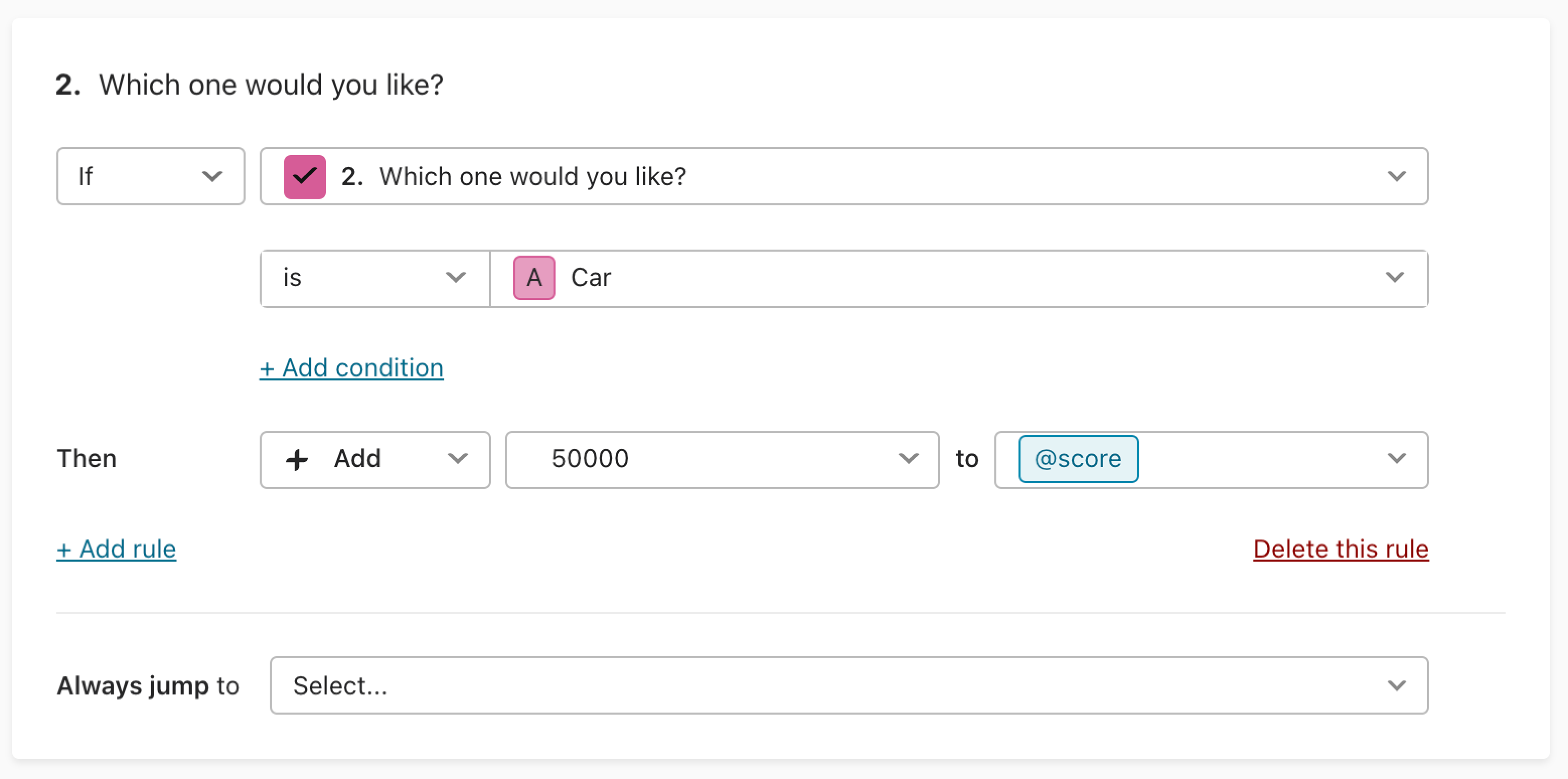Finally, you can create as many variables for your typeform as you want and reference them in calculations. You can even perform mathematical operations between variables. This article explains how to add and change variables.

When you’ve finished adding calculations to a question, remember to hit Save, and you'll be returned to the Create panel to continue building your typeform.

### Default score value

You can set a default value for your score in the Logic tab. Switch to Advanced Logic and click Variables: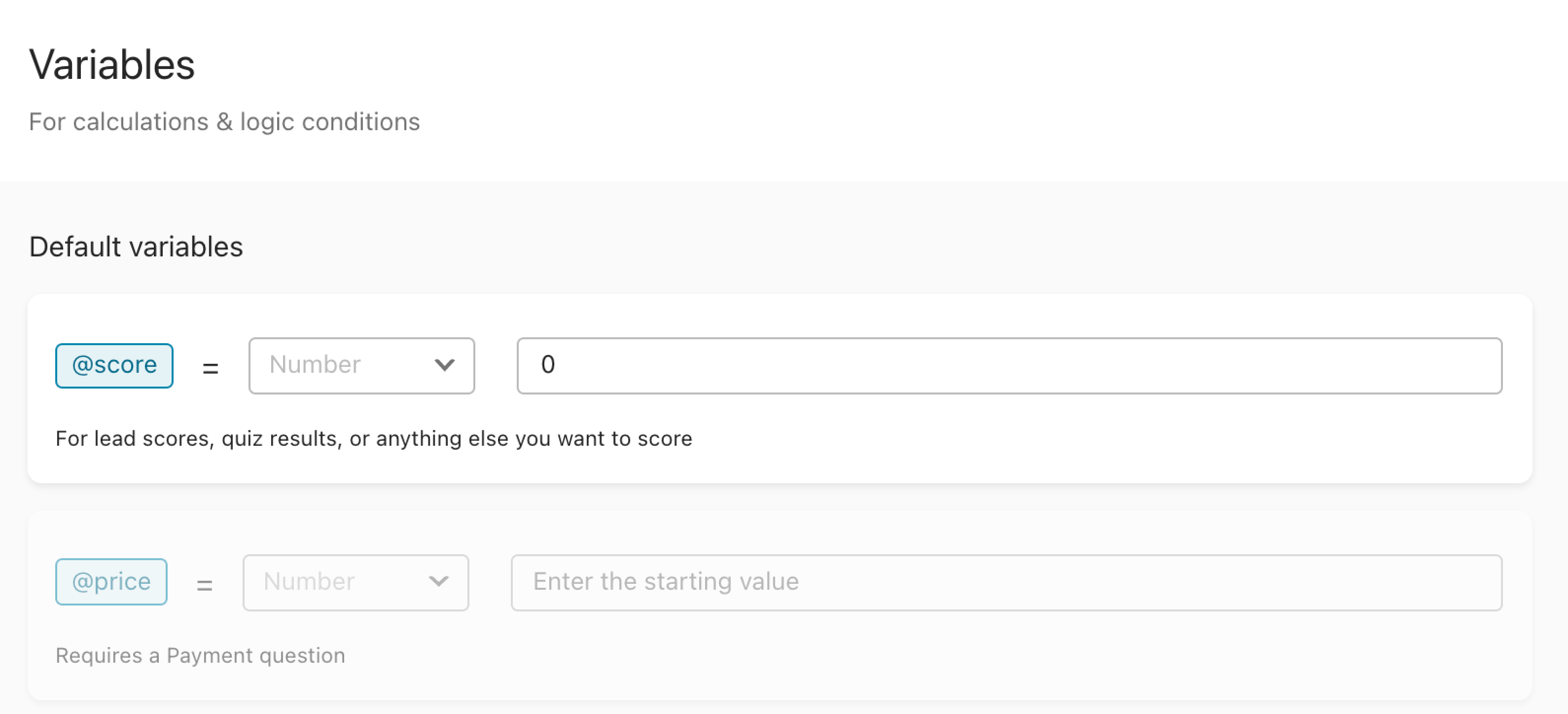The default value for score is initially set at 0. This means that everyone who responds to the typeform will have a score of 0 at the beginning of the form.

To change the default value, first set up at least one logical rule that changes respondents’ scores based on their answers. Once you’ve done that, you can set the default value to any number you like.

### Reference score values in your typeform

You can use Recall information to show score values anywhere in your typeform. For example, you might reference a respondent's final quiz score in the Ending screen.

In situations like the ones we've set up here, the typeform will show updated values after a respondent answers the questions that you've created rules for. In other words, if you've set up a rule for Question 2 that changes a variable's value, you can reference that variable in Question 3 to show its updated value.